Updating search results...

# 146 Results

View
Selected filters:
• Simulation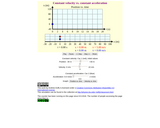Conditional Remix & Share Permitted
CC BY-SA
Rating
0.0 stars

This simulation shows the difference between Constant Velocity vs. Constant Acceleration هذه المحاكاة تبين الفرق بين السرعه الثابته والتسارع المستمر في الفيزياء

Subject:
Physics
Science
Material Type:
Simulation
Provider:
Boston University
Author:
Andrew Duffy
06/03/2019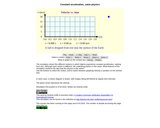Conditional Remix & Share Permitted
CC BY-SA
Rating
0.0 stars

The simulation shows five different motions in which objects experience constant acceleration, starting from rest. Although each motion is different, the underlying physics is the same. What features of the simulation reinforce the idea that the physics is the same?

Subject:
Physics
Science
Material Type:
Simulation
Provider:
Boston University
Author:
Andrew Duffy
06/03/2019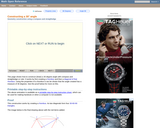Rating
0.0 stars

An interactive applet and associated web page that show how to construct a 30 degrees angle with a compass and straightedge. The animation can be single-stepped or run as a continuous movie. Applet can be enlarged to full screen size for use with a classroom projector. This resource is a component of the Math Open Reference Interactive Geometry textbook project at http://www.mathopenref.com.

Subject:
Geometry
Mathematics
Material Type:
Simulation
Provider:
Math Open Reference
Author:
John Page
05/07/2019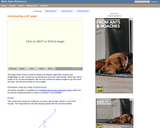Rating
0.0 stars

An interactive applet and associated web page that show how to construct a 45 degrees angle with a compass and straightedge. The animation can be single-stepped or run as a continuous movie. Applet can be enlarged to full screen size for use with a classroom projector. This resource is a component of the Math Open Reference Interactive Geometry textbook project at http://www.mathopenref.com.

Subject:
Geometry
Mathematics
Material Type:
Simulation
Provider:
Math Open Reference
Author:
John Page
05/07/2019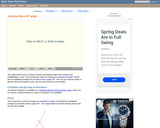Rating
0.0 stars

An interactive applet and associated web page that show how to construct a 60 degrees angle with a compass and straightedge. The animation can be single-stepped or run as a continuous movie. Applet can be enlarged to full screen size for use with a classroom projector. This resource is a component of the Math Open Reference Interactive Geometry textbook project at http://www.mathopenref.com.

Subject:
Geometry
Mathematics
Material Type:
Simulation
Provider:
Math Open Reference
Author:
John Page
05/07/2019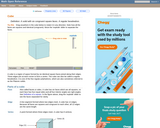Rating
0.0 stars

An interactive applet and associated web page that demonstrate the properties of a cube. A 3-D cube is shown in the applet which can be interactively manipulated using the mouse. Research has shown that some younger students have difficulty visualizing the parts of a 3D object that are hidden. To help with this, the student can rotate the cube in any axis simply by dragging it with the mouse. It can also be 'exploded' - where a slider gradually separates the faces to reveal the ones behind. The cube can also be made translucent so you see through it to the other side. Applet can be enlarged to full screen size for use with a classroom projector, and printed to make handouts. This resource is a component of the Math Open Reference Interactive Geometry textbook project at http://www.mathopenref.com.

Subject:
Geometry
Mathematics
Material Type:
Simulation
Provider:
Math Open Reference
Author:
John Page
05/07/2019Rating
0.0 stars

Students explore the volume of a box based on the amount of unit cubes that can fit inside of it in this student interactive, from Illuminations. They are prompted to come up with a rule for determining the volume of a box when its width, depth, and height are known.

Subject:
Geometry
Mathematics
Material Type:
Activity/Lab
Interactive
Simulation
Provider:
National Council of Teachers of Mathematics
Author:
Illuminations
02/26/2019Rating
0.0 stars

DASHlink is a virtual laboratory for scientists and engineers to disseminate results and collaborate on research problems in health management technologies for aeronautics systems. Managed by the Integrated Vehicle Health Management project within NASA's Aviation Safety program, the Web site is designed to be a resource for anyone interested in data mining, IVHM, aeronautics and NASA.

Subject:
Science
Material Type:
Lecture
Primary Source
Simulation
Provider:
NASA
07/11/2003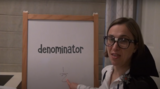Unrestricted Use
CC BY
Rating
0.0 stars

This lesson is Day 10 in a series of 12 lessons around fraction equivalences and comparisons.

This video lesson serves as a review of equpartitioning in order to determine the fractional part of an area or length. This is a necessary foundational skill for comparing and finding equivalent fractions.

Subject:
Mathematics
Material Type:
Activity/Lab
Interactive
Lesson
Simulation
Author:
Dawne Coker
06/25/2020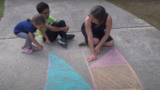Unrestricted Use
CC BY
Rating
0.0 stars

This lesson is Day 11 in a series of 12 lessons around fraction equivalences and comparisons.

This video lesson reviews parts of a whole, comparing fractions, and equivalent fractions. No materials are needed.

Subject:
Mathematics
Material Type:
Activity/Lab
Interactive
Lesson
Simulation
Author:
Dawne Coker
06/25/2020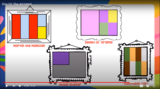Unrestricted Use
CC BY
Rating
0.0 stars

This lesson is Day 12 in a series of 12 lessons around fraction equivalences and comparisons.

Use your math skills to help detectives solve the crime. This video lesson reviews fractions of an area, fractions on a number line, and equivalent fractions. No materials are required.

Subject:
Mathematics
Material Type:
Activity/Lab
Interactive
Lesson
Simulation
Author:
Dawne Coker
06/25/2020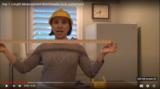Unrestricted Use
CC BY
Rating
0.0 stars

This lesson is Day 1 in a series of 5 lessons around U.S customary measurement.

This 3rd grade video lesson introduces the U.S. customary measurements for length: inches, feet, and yards. In this lesson, students will learn benchmarks (visuals) for measuring in inches, feet and yards when they don't have standard measurement tools available.

Subject:
Mathematics
Material Type:
Activity/Lab
Interactive
Lesson
Simulation
Author:
Dawne Coker
06/25/2020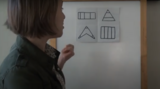Unrestricted Use
Public Domain
Rating
0.0 stars

This lesson is Day 1 in a series of 12 lessons around fraction equivalences and comparisons.

This video lesson encourages students to reason about and visualize fractions. The lesson focuses on the ideas that fractions are fair shares and that fractions can be compared. This lesson assumes that students have some initial experiences with fractions.

Subject:
Mathematics
Material Type:
Interactive
Lesson
Presentation
Simulation
Author:
Dawne Coker
06/25/2020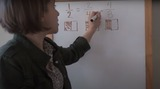Unrestricted Use
Public Domain
Rating
0.0 stars

This lesson is Day 2 in a series of 12 lessons around fraction equivalences and comparisons.

This video lesson uses paper folding to introduce the concept of equivalent fractions. To complete this lesson, make sure you have a square piece of paper (any size) and a crayon

Subject:
Mathematics
Material Type:
Activity/Lab
Interactive
Lesson
Simulation
Author:
Dawne Coker
06/25/2020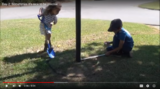Unrestricted Use
CC BY
Rating
0.0 stars

This lesson is Day 1 in a series of 5 lessons around U.S customary measurement.

This lesson focuses on measuring lengths to the nearest fourth of a inch, and discusses when it's necessary to find a precise measurement versus an estimate.

Subject:
Mathematics
Material Type:
Activity/Lab
Interactive
Lesson
Simulation
Author:
Dawne Coker
06/25/2020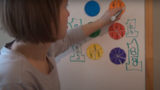Unrestricted Use
CC BY
Rating
0.0 stars

This lesson is Day 2 in a series of 12 lessons around fraction equivalences and comparisons.

This video lesson introduces the idea that fractions can be used to represent 1 whole. To engage in this lesson, students may use virtual circle models from Toy Theater (https://toytheater.com/fraction-circles/)

Subject:
Mathematics
Material Type:
Activity/Lab
Interactive
Lesson
Simulation
Author:
Dawne Coker
06/25/2020Unrestricted Use
CC BY
Rating
0.0 stars

This lesson is Day 3 in a series of 5 lessons around U.S customary measurement.

This video lesson explores the use of appropriate units for measuring length. Additionally, students will be asked to solve single-step problems involving lengths of distances. No materials are needed for this lesson.

Subject:
Mathematics
Material Type:
Activity/Lab
Interactive
Lesson
Simulation
Author:
Dawne Coker
06/25/2020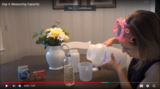Unrestricted Use
CC BY
Rating
0.0 stars

This lesson is Day 1 in a series of 5 lessons around U.S customary measurement.

This video lesson introduces U.S. customary units for measuring capacity (cups, pints, quarts, and gallons). Students examine benchmarks for each unit, and then apply their knowledge of capacity to make lava lamps. To make a lava lamp, students will need: 1 quart-size empty water bottle, 2 cups vegetable oil, 1 cup water, food coloring, 1 alka-seltzer tablet. (All measurements are approximate and may be adjusted based on the capacity of the water bottle).

Subject:
Mathematics
Material Type:
Activity/Lab
Interactive
Lesson
Simulation
Author:
Dawne Coker
06/25/2020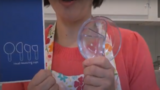Unrestricted Use
CC BY
Rating
0.0 stars

This lesson is Day 4 in a series of 12 lessons around fraction equivalences and comparisons.

This video lesson looks at how we use fractions in the kitchen. Students are challenged to use circle models to find equivalent fractions for a recipe. Students may use virtual circe models from the Toy Theater (https://toytheater.com/fraction-circles/).

Subject:
Mathematics
Material Type:
Activity/Lab
Interactive
Lesson
Simulation
Author:
Dawne Coker
06/25/2020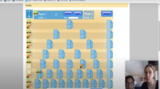Unrestricted Use
CC BY
Rating
0.0 stars

This lesson is Day 2 in a series of 12 lessons around fraction equivalences and comparisons.

This game may be played with one or two people. It reviews fractions on a number line, adding fraction with like denominators, and equivalent fractions. For an extra challenge, you can even break up your moves across multiple tracks (e.g., if your card reads 1/2, you could move 1/4 and 2/8).

https://www.nctm.org/Classroom-Resources/Illuminations/Interactives/Playing-Fraction-Track/

Subject:
Mathematics
Material Type:
Activity/Lab
Interactive
Lesson
Simulation
Author:
Dawne Coker